Select Page

# Principle Stresses and Strains

Principal Stresses and Strains

Contents

Recall the concept of Critical Point and Critical Section 1

Recall the condition of Plane Stress 1

Recall the Matrix of Stress Tensor 2

Define Principal Stress and Principal Plane 3

Recall the Plane of Maximum Shear Stress 5

Recall the concept of Pure Shear Stress Plane 6

Recall and calculate the Stresses developed due to Direct Stress or Uniaxial Stress condition 6

Recall and calculate the Stresses developed due to Biaxial with Zero Shear Stress condition 7

Recall and calculate the Stresses developed due to Pure shear stress condition 8

Recall and calculate the Stresses developed due to Biaxial with Shear Stress condition 9

Recall Sum of Normal Stress on Two Mutually Perpendicular Planes 10

Describe and calculate Principal Stresses and its Planes 11

Describe and calculate Maximum Shear Stresses and their Planes 12

Describe and calculate Normal Stress on the Plane of Maximum Shear Stress 13

Recall the concept of Mohr’s Circle 14

Draw the Mohr’s Circle for the following State of Stress i. For Uniaxial Stress ii. For Bi-axial with Zero Shear Stress iii. For Pure Shear Stress iv. For Bi-axial with Shear Stress 16

Recall State of Strain and Strain Tensor 18

Recall and calculate the Normal Strain in Oblique Planes 19

Explain and calculate the Shear Strains in Oblique Planes 20

Determine the Principal Strain and its Planes 21

Determine Maximum Shear Strain and its Planes 22

Determine the relation between Principal Stresses and Principal Strains 23

Recall the concept of Strain Rosettes 24

Recall and calculate the Strain Energy in Three-Dimensional Stress System 25

Recall and calculate the Shear Strain Energy in Three-Dimensional Stress System 25

# Recall the concept of Critical Point and Critical Section

In structural engineering, a critical point refers to a location along a beam or structure where the internal forces (i.e., bending moment, shear force, axial force) are at a maximum or minimum. At these critical points, the stress and strain are also at maximum or minimum values. Critical points can occur at different locations depending on the type of loading applied to the structure.

On the other hand, a critical section refers to a section of a beam or structure that is subjected to the highest internal forces, and hence, the maximum stress and strain. The critical section is the location where failure is most likely to occur. It is important to identify the critical section of a structure in order to ensure that the design is safe and can withstand the applied loads.

The critical section for bending moment is usually at the point where the bending moment is maximum, while for shear force, it is usually at the point where the shear force is maximum. For axial force, the critical section is usually located where the force is applied, such as the mid-span of a beam. The identification of critical sections is a key aspect of structural design and analysis, as it helps to determine the strength and safety of a structure.

# Recall the condition of Plane Stress

Plane stress is a condition where a two-dimensional (2D) body or material is subjected to loads or forces that are confined to a single plane, and the stresses acting on the body are non-zero only within that plane. In other words, the stresses acting perpendicular to the plane of loading are negligible.

The condition of plane stress is often used to analyze and design thin plates, such as metal sheets, that are subjected to loads and forces in a plane. The stresses acting within the plane are typically calculated using the equations of stress and strain that are derived from the theory of elasticity.

The three components of stress that can exist in a plane stress condition are normal stress, shear stress, and bending stress. Normal stress is the stress that acts perpendicular to the plane of loading, while shear stress is the stress that acts tangential to the plane of loading. Bending stress is the stress that results from the curvature of the plate, and it is a combination of normal and shear stress.

The condition of plane stress is important for engineers and designers because it allows them to simplify the analysis of complex structures, such as aircraft wings and bridges, and to make more accurate predictions about the strength and behaviour of the structures under load.

# Recall the Matrix of Stress Tensor

The stress tensor is a mathematical representation of the stress state at a point in a material or structure. It is a second-order tensor that relates the stress components to the coordinate axes of a Cartesian coordinate system.

In a three-dimensional Cartesian coordinate system, the stress tensor is represented by a 3×3 matrix of stress components. The matrix has six independent stress components, which are usually denoted by the symbols σxx, σyy, σzz, σxy, σxz, and σyz. These stress components represent the normal and shear stresses acting on the three orthogonal planes in the material.

The stress tensor matrix can be written as: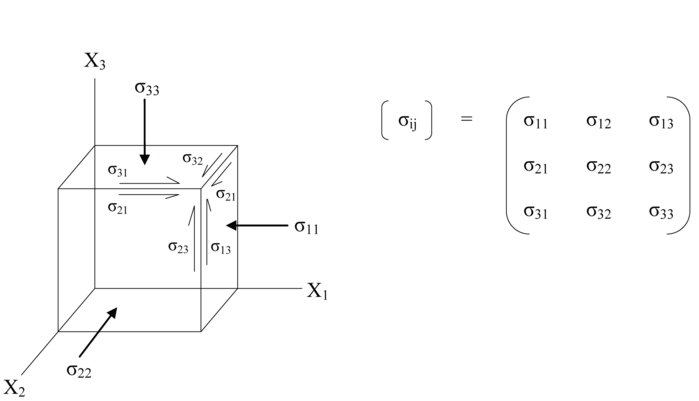The diagonal elements of the matrix represent the normal stresses acting on the x, y, and z planes, while the off-diagonal elements represent the shear stresses acting on the x-y, x-z, and y-z planes.

The stress tensor matrix is an important tool in solid mechanics and structural analysis, as it provides a way to calculate the stresses and strains in complex structures under different loading conditions. It is used in conjunction with other mathematical and computational techniques, such as the finite element method, to analyze and design structures that can withstand different types of loads and forces.

# Define Principal Stress and Principal Plane

In solid mechanics, principal stress and principal plane are important concepts that help to simplify the analysis of the stress state in a material or structure.

Principal stresses are the maximum and minimum normal stresses acting on a given point in a material or structure. The principal stresses are always mutually orthogonal to each other and are represented by the symbols σ1 and σ2, where σ1 is the maximum principal stress and σ2 is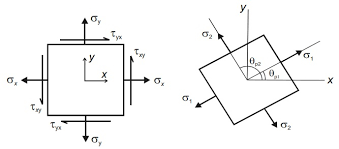the minimum principal stress.

The principal plane is the plane on which the shear stress is zero and the normal stress is either σ1 or σ2. This plane is perpendicular to the direction of the maximum principal stress (σ1) and is known as the plane of maximum shear stress.

The concept of principal stress and plane is used to simplify the stress state in a material or structure and to determine the critical stresses that can cause failure. For example, if the material is ductile, yielding will occur when the maximum principal stress exceeds the yield stress. If the material is brittle, failure will occur when the maximum principal stress exceeds the tensile strength.

The calculation of principal stresses and planes can be done using mathematical methods such as the Mohr’s circle or the eigenvalue method. The principal stress and plane can also be visualised using graphical methods, such as 3D stress ellipsoids, which provide a clear understanding of the stress state in the material.

# Recall the Plane of Maximum Shear Stress

In solid mechanics, the plane of maximum shear stress is a plane where the shear stress is maximum among all planes passing through the given point. This plane is perpendicular to the direction of the maximum principal stress and is called the plane of maximum shear stress.

The concept of the plane of maximum shear stress is often used in the analysis of the stress state in a material or structure. In this analysis, it is assumed that the material will fail when the maximum shear stress exceeds the shear strength of the material.

The calculation of the plane of maximum shear stress can be done using mathematical methods such as the Mohr’s circle or the stress transformation equations. In the Mohr’s circle method, the stresses are plotted on a circle to determine the principal stresses and the plane of maximum shear stress. In the stress transformation equations method, the stresses are transformed into a different coordinate system to determine the principal stresses and the plane of maximum shear stress.

The plane of maximum shear stress is important in the design of structures that are subjected to bending, torsion, or shear stresses. By knowing the location of the plane of maximum shear stress, engineers can design structures that are strong enough to withstand the applied loads without failure.

Overall, understanding the concept of the plane of maximum shear stress is crucial in the field of solid mechanics, as it plays a significant role in the analysis and design of structures under various loading conditions.

# Recall the concept of Pure Shear Stress Plane

Pure shear stress is a type of stress that occurs when two opposing forces act parallel to each other but in opposite directions. This creates a state of stress where the stress on one face of the material is equal and opposite to the stress on the other face. The plane where pure shear stress acts is known as the pure shear stress plane.

In this plane, the stresses acting on it are purely shear stresses, meaning there is no normal stress acting on it. The normal stress on this plane is zero. This results in a unique stress state in which the material experiences deformation in one direction and contraction in another direction perpendicular to it.

Pure shear stress can be visualised as taking a material and pulling it in opposite directions along parallel planes. The resulting stress on the material will be pure shear stress, which can cause it to undergo shearing deformation.

The pure shear stress plane is important in the analysis of materials and structures subjected to shear stresses. It is often used in the study of metal forming processes, where the material undergoes plastic deformation due to shear stresses.

Overall, understanding the concept of the pure shear stress plane is important in the field of solid mechanics as it provides an insight into the behaviour of materials under shear stresses and can aid in the design of structures that can withstand these stresses without failure.

# Recall and calculate the Stresses developed due to Direct Stress or Uniaxial Stress condition

Direct stress or uniaxial stress is a type of stress where the force is applied in a single direction perpendicular to the surface of the object. This type of stress is commonly observed in structures and materials subjected to tensile or compressive loads.

The formula to calculate the stress due to direct stress or uniaxial stress condition is:

σ = F/A

Where:

• σ is the stress in the material
• F is the force applied to the material
• A is the cross-sectional area of the material perpendicular to the force

If the force is tensile, the stress is known as tensile stress and it is positive. If the force is compressive, the stress is known as compressive stress and it is negative.

Direct stress or uniaxial stress is a simple type of stress that can be easily calculated using basic mathematical principles. It is important in the analysis and design of various structures and materials to ensure they can withstand the loads and stresses to which they will be subjected.

# Recall and calculate the Stresses developed due to Biaxial with Zero Shear Stress condition

In a biaxial stress condition with zero shear stress, the stress state of an object is characterized by two mutually perpendicular stresses, σ x and σy, with no shear stress. This type of stress condition is also known as pure axial stress.

The formula to calculate the stress developed in an object subjected to biaxial stress with zero shear stress is:

σmax = ( x + σ y)/2 + sqrt((( x – σ y)/2)2 + τ xy2)

Where:

• σmax is the maximum principal stress
• σx and σy are the stresses in the x and y directions, respectively
• τxy is the shear stress

The shear stress, τxy, is zero in this case.

The maximum principal stress, σmax, is the maximum stress value experienced by the object. The direction of the maximum principal stress is called the direction of principal stress.

Biaxial stress with zero shear stress is common in the analysis of thin-walled structures, such as pressure vessels, which are often subjected to internal pressure. In these cases, the stress state of the vessel is assumed to be biaxial with zero shear stress, and the above equation is used to calculate the maximum principal stress in the vessel. The calculated stress is then compared to the yield stress of the material to ensure that the vessel is strong enough to withstand the internal pressure.

# Recall and calculate the Stresses developed due to Pure shear stress condition

When a body is subjected to two equal and opposite forces acting parallel to its cross-sectional area, the body experiences a shear stress. The magnitude of shear stress acting on the cross-sectional area is known as the pure shear stress. Pure shear stress is an important concept in the field of mechanics of materials and is used to calculate the maximum shear stress that can be developed in a material under different loading conditions.

The formula for calculating the magnitude of pure shear stress is:

τ = (F/A) or τ = (VQ/It)

where,

τ = pure shear stress

F = applied force

A = cross-sectional area

V = shear force

Q = first moment of area

t = thickness of the section

I = moment of inertia

In a rectangular section, the pure shear stress develops along the plane inclined at 45 degrees to the x-axis and the y-axis. At this plane, both the principal stresses are equal and opposite, and the magnitude of the pure shear stress is equal to half the difference between the magnitudes of the principal stresses.

The maximum shear stress developed in a material under any loading condition can be found by adding the pure shear stress to half the difference between the maximum and minimum principal stresses. The maximum shear stress is important in the design of structural members and is used to determine the material’s ability to resist shear stress.

# Recall and calculate the Stresses developed due to Biaxial with Shear Stress condition

In a biaxial state of stress with shear stress, the normal stresses and shear stresses act in different planes. The normal stresses are acting in the direction perpendicular to the plane of shear stress. The maximum and minimum principal stresses are determined by adding or subtracting the shear stress from the average normal stress. The formula to calculate the principal stresses under this condition is:

σ1,2 = (σx + σy) / 2 ± [(σx – σy) / 2]2 + τxy2

where,

σ1 and σ2 are the maximum and minimum principal stresses,

σx and σy are the normal stresses in the x and y directions respectively, and

τxy is the shear stress acting on the plane.

The angle that the plane of maximum shear stress makes with the x-axis can be calculated using the formula:

tan 2θ = 2 τxy / (σx – σy)

The maximum shear stress can be determined using the formula:

τmax = (σx – σy) / 2

where τmax is the maximum shear stress.

Once the maximum and minimum principal stresses and the plane of maximum shear stress are known, the stresses acting on any plane can be determined using Mohr’s circle.

# Recall Sum of Normal Stress on Two Mutually Perpendicular Planes

The sum of normal stresses on two mutually perpendicular planes is a fundamental concept in the study of stress analysis. This concept is based on the idea that an element of material in a structure can be considered to be in a state of equilibrium if the sum of the forces acting on it in all directions is equal to zero. In the context of stress analysis, this principle can be expressed as the sum of normal stresses on two mutually perpendicular planes being equal to the applied stress.

When a material is subjected to an external force, it experiences stresses that are distributed throughout its structure. These stresses can be resolved into normal stresses, which act perpendicular to the planes on which they are applied, and shear stresses, which act parallel to these planes. The sum of the normal stresses acting on any two mutually perpendicular planes can be calculated using the following formula:

σ = σ x + σ y

Where σ is the sum of the normal stresses, σx is the normal stress acting on one plane, and σy is the normal stress acting on a plane perpendicular to the first plane. The sum of the normal stresses is important in the analysis of materials, as it can help to determine the maximum stresses that a material can withstand before it fails.

# Describe and calculate Principal Stresses and its Planes

Principal stress is the maximum and minimum normal stress in a given stress state, and they occur on planes perpendicular to each other. These stresses are important as they determine the failure of materials under loading.

To calculate the principal stresses and their planes, we need to first obtain the stresses acting on the element. We can represent these stresses by a stress matrix which can be in the form of a 2×2 or 3×3 matrix, depending on the dimensions of the element. For example, in a two-dimensional plane stress problem, the stress matrix is given by:

x, τxy]

xy, σy]

where σx and σy are normal stresses acting in the x and y directions, respectively, and τxy is the shear stress acting on the xy plane.

To calculate the principal stresses and their planes, we start by finding the roots of the characteristic equation of the stress matrix, which is given by:

λ2 – (σx + σy)λ + (σx σy – τxy2) = 0

where λ is the eigenvalue of the stress matrix. The two roots of this equation are the principal stresses, σ1 and σ2, given by:

σ1,2 = (σx + σy)/2 ± √[(σx – σy)2/4 + τxy2]

The corresponding principal planes are given by the eigenvectors of the stress matrix. These are the directions in which the principal stresses act. We can calculate the eigenvectors by solving the system of linear equations:

x – σ1 τxy] [n1] = 

xy σy – σ1] [n2] 

where n1 and n2 are the components of the eigenvector.

Once we have found the principal stresses and their planes, we can determine whether the material will fail under the given loading conditions. If either of the principal stresses exceeds the yield strength of the material, then the material will fail. We can also calculate the maximum shear stress acting on the element, which is given by:

τmax = (σ1 – σ2)/2

This is the maximum shear stress acting on the element, and it occurs on a plane at 45 degrees to the principal planes.

# Describe and calculate Maximum Shear Stresses and their Planes

In solid mechanics, the maximum shear stress theory or Tresca’s theory states that yielding will occur in a material when the maximum shear stress at any point in the material exceeds the shear strength of the material.

To calculate the maximum shear stress and its planes, we need to first find the principal stresses and their planes. The principal stresses are the normal stresses acting on the planes, where there is no shear stress. We can find the principal stresses by solving the characteristic equation of the stress tensor.

Once we have found the principal stresses, we can determine the maximum shear stress by taking half of the difference between the two principal stresses. The maximum shear stress occurs on a plane that is inclined at 45 degrees to the planes of the principal stresses. The orientation of the maximum shear stress plane can be found by drawing a circle of stress on a Mohr’s circle diagram, where the radius of the circle represents the average stress and the center of the circle represents the origin of the stress tensor. The tangent to the circle at the point where it intersects the 45-degree line represents the orientation of the maximum shear stress plane.

To calculate the maximum shear stress, we use the formula:

Maximum Shear Stress = 1/2 (σ1 – σ2)

where σ1 and σ2 are the principal stresses. The planes on which the maximum shear stress occurs are perpendicular to each other and to the plane of the principal stresses.

Calculating the maximum shear stress and its planes is important in determining the failure of a material, as it is the criterion for failure according to Tresca’s theory.

# Describe and calculate Normal Stress on the Plane of Maximum Shear Stress

In mechanics of materials, normal stress on the plane of maximum shear stress is a critical parameter in determining the failure of a material under loading. The maximum shear stress theory states that the failure of a material occurs when the maximum shear stress exceeds a certain value, which is the shear strength of the material.

When a material is subjected to an arbitrary state of stress, the maximum shear stress and the normal stress on the plane of maximum shear stress can be calculated using the following equations:

Maximum Shear Stress:

τmax = (σ1 – σ2) / 2

where σ1 and σ2 are the principal stresses, and τmax is the maximum shear stress.

Normal Stress on the Plane of Maximum Shear Stress:

σn = (σ1 + σ2) / 2

where σn is the normal stress on the plane of maximum shear stress.

Once these values are calculated, they can be compared with the shear strength and normal stress limits of the material to determine whether the material will fail or not.

It is important to note that these calculations assume that the material is isotropic and homogeneous, and that the stress state is static and unchanging. In more complex situations, such as those involving dynamic loading or non-homogeneous materials, more advanced analysis techniques may be required.

# Recall the concept of Mohr’s Circle

Mohr’s Circle is a graphical method used to find the normal and shear stresses acting on any inclined plane in a 2D stress state. The method involves plotting the normal and shear stresses acting on the two mutually perpendicular planes on the x-axis and y-axis, respectively. The resulting plot is a circle, known as Mohr’s Circle.

The center of the circle represents the average normal stress, and the radius of the circle is equal to half the difference between the maximum and minimum normal stresses. The angle between the x-axis and the line joining the center of the circle and any point on the circumference of the circle is equal to twice the angle between the plane and the x-axis.

Mohr’s Circle is used to determine the principal stresses and their planes, the maximum shear stress and its plane, and the normal stress acting on the plane of maximum shear stress. It is also used to determine the stresses acting on a plane at any arbitrary angle to the x and y axes.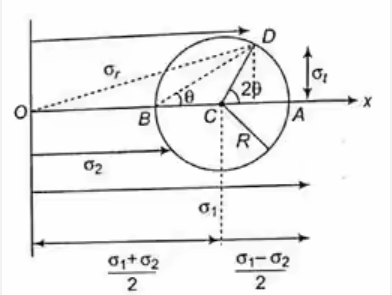Mohr’s Circle is widely used in the field of structural engineering and mechanics to analyze the stresses acting on various structural members and components, such as beams, columns, and plates. It is a powerful tool for visualizing and understanding stress states and their effects on different materials and structures.

# Draw the Mohr’s Circle for the following State of Stress i. For Uniaxial Stress ii. For Bi-axial with Zero Shear Stress iii. For Pure Shear Stress iv. For Bi-axial with Shear Stress

Mohr’s Circle is a graphical representation of the stress state for a two-dimensional plane stress problem. The stress state is represented by plotting the normal stress (σ) on the x-axis and the shear stress (τ) on the y-axis. The center of the circle represents the average normal stress, and the radius of the circle represents the maximum shear stress.

The Mohr’s Circle can be drawn for different states of stress as follows:

i. For Uniaxial Stress:

In the case of uniaxial stress, the Mohr’s circle is a point on the x-axis. The normal stress is equal to the applied stress, and there is no shear stress.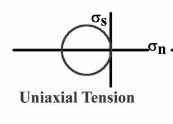ii. For Bi-axial with Zero Shear Stress:

In the case of bi-axial stress with zero shear stress, the Mohr’s circle is a horizontal line passing through the point (σx, 0) on the x-axis. The two principal stresses are σx and σy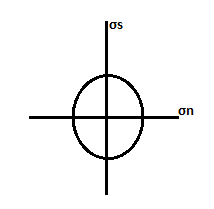iii. For Pure Shear Stress:

In the case of pure shear stress, the Mohr’s circle is a circle with the center at the origin (0, 0) and the radius equal to the magnitude of the shear stress.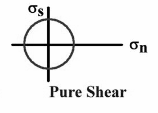iv. For Bi-axial with Shear Stress:

In the case of bi-axial stress with shear stress, the Mohr’s circle is a circle with the center at the point ((σx + σy)/2, 0) and the radius equal to half the difference between the two principal stresses.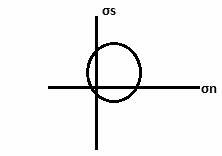To draw the Mohr’s Circle, we first mark the point (σx, τxy) and (σy, -τxy) on the σ-τ plane. These two points represent the stress state on two perpendicular planes. Then, we draw a circle passing through these two points, and the center of the circle represents the average normal stress. The two intersections of the circle with the x-axis represent the principal stresses, and the radius of the circle represents the maximum shear stress.

In conclusion, the Mohr’s Circle is a powerful tool for visualizing and analyzing two-dimensional stress states. It provides a graphical representation of the stress state, which can be used to determine the principal stresses and the maximum shear stress.

# Recall State of Strain and Strain Tensor

In mechanics, strain refers to the degree of deformation an object experiences as a result of stress applied to it. Strain is measured by the ratio of the change in length to the original length of an object, and it is a dimensionless quantity.

The strain tensor is a mathematical representation of the degree of deformation in a solid body subjected to stress. It is a second-order tensor that characterises the change in shape of a body as a result of applied stress. The strain tensor consists of six components, three normal strains and three shear strains, which are represented as a matrix.

The normal strains represent the elongation or contraction of the material in the direction perpendicular to the stress axis. The shear strains represent the changes in angle between two perpendicular lines in the material.

The strain tensor can be used to calculate the deformation, displacement, and stress in a material under different loading conditions. It is a key component in the study of solid mechanics and materials science.

# Recall and calculate the Normal Strain in Oblique Planes

When a structure is subjected to stress, it experiences deformation, which is quantified as strain. Strain is defined as the ratio of the change in length to the original length of the material. The strain tensor represents the strain state of a structure under stress. It is a second-order tensor that has nine components.

When a body experiences deformation, its original shape changes, and the change in length is not necessarily the same in all directions. This deformation results in different strains in different directions. The normal strain in an oblique plane is the change in length of the material in the direction perpendicular to the plane, divided by the original length of the material in that direction.

To calculate normal strain in oblique planes, consider a rectangular element of length dx, dy, and dz in the x, y, and z directions, respectively. The normal strain in the x direction is given by the change in length in the x direction divided by the original length in the x direction. Similarly, the normal strain in the y and z directions can be calculated.

The strains in the oblique directions are related to the normal strains using the strain tensor. The strain tensor is symmetric, which means that the strains in the x direction due to the y deformation are equal to the strains in the y direction due to the x deformation. Thus, the strain tensor contains only six unique components.

In summary, to calculate normal strain in oblique planes, we use the strain tensor, which represents the strain state of a structure under stress. We calculate the normal strain in the x, y, and z directions and relate the strains in oblique directions to the normal strains using the strain tensor.

# Explain and calculate the Shear Strains in Oblique Planes

Shear strain is the angular distortion caused by shear stress. In a general case, for a given element in an oblique plane, the shear strain can be described by two distinct components.

The first component of the shear strain, known as the engineering shear strain, γ, is the tangent of the angle between the deformed shape and the undeformed shape. Mathematically, it is given by:

γ = tan θ

where θ is the angle through which the right angle of the undeformed element is rotated to reach the corresponding right angle of the deformed element.

The second component of the shear strain, known as the natural shear strain, ε, is equal to the difference in strains in two perpendicular directions divided by two. Mathematically, it can be given by:

ε = (εx – εy) / 2

where εx and εy are the normal strains in the two perpendicular directions.

To calculate the shear strain for a given plane, we need to first determine the normal strains and the shear stresses acting on the plane. Once these values are determined, the shear strain can be calculated using the formulas mentioned above.

# Determine the Principal Strain and its Planes

The principal strain is defined as the maximum and minimum normal strains experienced by an element in the Cartesian coordinate system. The planes on which the principal strains act are called principal planes. The magnitude of the principal strains is equal to the eigenvalues of the strain tensor, and the orientation of the principal planes is given by the eigenvectors of the strain tensor.

To determine the principal strains and their planes, we need to find the eigenvalues and eigenvectors of the strain tensor. The steps involved in finding the principal strains and their planes are:

1. Form the strain tensor using the strain components in the Cartesian coordinate system.
2. Find the characteristic equation of the strain tensor by subtracting λI (where λ is an eigenvalue and I is the identity matrix) from the strain tensor and taking its determinant.
3. Solve the characteristic equation to find the eigenvalues of the strain tensor.
4. For each eigenvalue, find the corresponding eigenvector by solving the system of equations (strain tensor – λI)eigenvector = 0.
5. The eigenvalues represent the magnitudes of the principal strains, and the eigenvectors represent the directions of the principal planes.
6. If the principal strains are both positive, the principal planes are tensile; if both are negative, they are compressive; if one is positive and the other is negative, they form a shear plane.
7. The angle between the principal plane and the x-axis can be calculated as tan⁻¹(v/u), where u and v are the x and y components of the eigen vector, respectively.
8. The principal strain on the principal plane can be calculated using the corresponding eigenvalue.

In summary, the principal strains and their planes represent the maximum and minimum normal strains experienced by an element, and can be determined by finding the eigenvalues and eigenvectors of the strain tensor.

# Determine Maximum Shear Strain and its Planes

The maximum shear strain is the maximum difference in the strain between two mutually perpendicular planes. When the strain on two mutually perpendicular planes is equal to zero, the shear strain on a third plane perpendicular to them is the maximum. The magnitude of the maximum shear strain is half of the difference between the principal strains, and its direction is at an angle of 45 degrees to the principal plane with the larger strain.

To calculate the maximum shear strain, we can use the following formula:

γmax = (ε1 – ε2) / 2

where ε1 and ε2 are the principal strains. The angle at which the maximum shear strain occurs can be calculated using the following formula:

θ = 0.5 * tan⁻¹(2γxy / (ε1 – ε2))

where γxy is the shear strain in the plane perpendicular to the maximum shear strain plane.

The maximum shear strain occurs on the plane that is at 45 degrees to the plane of the larger principal strain. The plane of maximum shear strain can be found by rotating the plane of the larger principal strain by 45 degrees in the direction of the smaller principal strain.

# Determine the relation between Principal Stresses and Principal Strains

The relation between the principal stresses and principal strains is a fundamental concept in the mechanics of materials. Principal stresses are the normal stresses that act on the planes that are perpendicular to the principal planes, while principal strains are the normal strains that occur on these planes.

The relation between them can be obtained using the equation:

ε11 = ε22 = ε33

where σ1, σ2, and σ3 are the principal stresses and ε1, ε2, and ε3 are the principal strains. This equation states that the ratio of the strain to the stress is constant for all the principal planes.

The above equation can be rearranged to express the principal strains in terms of the principal stresses as:

ε1 = (σ1/E) – ν(σ2/E) – ν(σ3/E)

ε2 = -ν(σ1/E) + (σ2/E) – ν(σ3/E)

ε3 = -ν(σ1/E) – ν(σ2/E) + (σ3/E)

where E is the modulus of elasticity of the material and ν is the Poisson’s ratio.

The above relations help us to find the values of principal strains, given the values of principal stresses, and vice versa. This is useful in many engineering applications, especially when we are dealing with complex stress and strain states.

In summary, the relation between principal stresses and principal strains is a critical concept in mechanics of materials, and it is essential to understand this relationship to analyze the behaviour of materials under different loading conditions.

# Recall the concept of Strain Rosettes

Strain rosettes are instruments used to measure strain in three dimensions. They are made up of three linear strain gauges arranged in a specific way to measure the strains in different directions. Strain rosettes are useful for measuring strains that occur in complex situations, where the strains in different directions may be significantly different.

The three linear strain gauges are mounted on a flat surface or a curved surface of the object being measured. The three gauges are arranged in such a way that they measure the strain in three perpendicular directions. The gauges are attached to a backing material, such as paper or plastic, which is then attached to the object being measured.

When a strain is applied to the object, each of the gauges will measure a different strain, and the readings can be used to calculate the magnitude and direction of the strain. The readings from the three gauges are combined to give the total strain in three dimensions, which can be used to determine the orientation of the strain, the magnitude of the strain, and the direction of the principal strains.

Strain rosettes are commonly used in the field of mechanical engineering, particularly in materials testing, and they are also used in geotechnical and structural engineering. They are also useful in testing the effectiveness of various materials, such as concrete or metal, to see how they perform under different types of strain.

# Recall and calculate the Strain Energy in Three-Dimensional Stress System

In a three-dimensional stress system, the deformation of an object can be described by a strain tensor, which is a second-order symmetric tensor that contains six independent components. The strain tensor is related to the stress tensor through the elastic modulus of the material. The elastic modulus relates the stresses to the strains and is a measure of the stiffness of the material.

The strain energy is a measure of the work required to deform an object under the influence of external forces. It is defined as the integral of the product of the stress and strain over the volume of the object. The strain energy density is defined as the strain energy per unit volume.

To calculate the strain energy, we first need to calculate the strain tensor from the stress tensor using the elastic modulus of the material. We then calculate the strain energy density using the equation:

Strain energy density = 1/2 * stress tensor * strain tensor

Finally, we integrate the strain energy density over the volume of the object to obtain the total strain energy.

The strain energy is an important parameter in the design and analysis of structures and materials. It can be used to predict the behaviour of a material under different loading conditions and to compare the performance of different materials.

# Recall and calculate the Shear Strain Energy in Three-Dimensional Stress System

Shear strain energy is the energy stored in a material due to the shear strain. It is a measure of the elastic energy stored in a solid when it is subjected to shear stress. Shear strain energy is also known as the complementary or deviatoric strain energy. This energy is independent of the volume change due to the deformation of the material.

The shear strain energy per unit volume can be calculated using the following equation:

2 / 2E × V

The shear modulus G is related to the Young’s modulus E and the Poisson’s ratio μ through the following equation:

Y=2G(1+μ)

where E is the Young’s modulus and $\nu$ is the Poisson’s ratio.

The above equation shows that the shear strain energy depends on the shear modulus of elasticity and the normal and shear strains. The shear strain energy is a measure of the work done on the material during the deformation process. It is an important parameter in the study of the mechanical behaviour of materials under load.